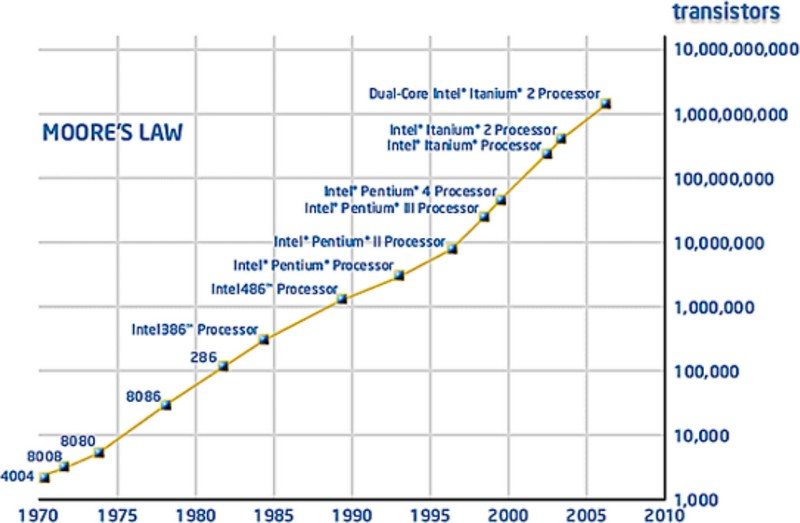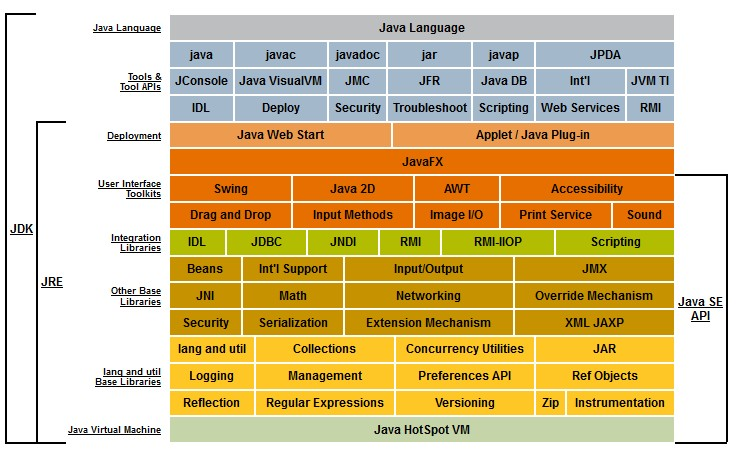# Float32 - 32-bit IEEE float (Single Precision)

32-bit IEEE float is a floating-point number encoded on 32 bit.

float32 is also known simply as float

## Implementation

• Sql: Real, Float

## Documentation / Reference

Discover More32 bits long word

32 bits long wordComputer - Float Number

FLOAT is short name for the float 32, a floating point number with a precision 32-bit.Computer - Floating-point (Representation and usage)

Computer representations of floating point numbers typically use a form of rounding to significant figures, but with binary numbers. The number of correct significant figures is closely related to the...Computer Storage - Integer Data Type (Binary Data)

In computer, integer are stored in word from 8 to 64 bit. Because CPU manipulates integer data type, they are also sometime called binary data type. Bit Length Two's complement signed Unsigned Float...Java - Float DataType

Float datatype in Java implements the 32-bit precision IEEE 754 floating point. Example of InitializationJava - Number

in Java. The abstract class Number is the superclass of platform classes representing numeric values that are convertible to the primitive types byte, double, float, int, long, and short. The...
Javascript - Byte Array (ArrayBuffer / DataView)

To manipulate a byte array, in javascript, you have: a ArrayBuffer that represents an array: of fixed amount of bytes (one element = 1 byte) of binary element known as typed object : Int8 / Uint8:...SQL - Data Type

The Data Type of a column in SQL. They are defined in the ANSI/ISO SQL standard but may vary from sql engine (database) to another. The below table shows you the history of the data type in the ANSI...SQL - Float

Float is a sql datatype is floating point number implemented as single precision float (32 bit) where: n is the number of bits that are used to store the mantissa n value Precision Storage...